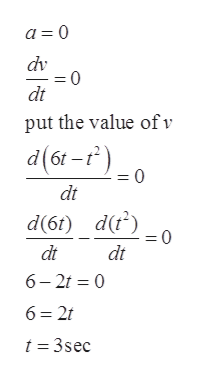# The velocity as a function of time is given as v(t) = 6t−t2 in m/s and t in seconds (s). Consider the time interval from t=0 tot = 12A:Find the time after t=0 when the particle changes direction.B:Find the time before which the acceleration is positive.C:What is the displacement of the object in the time interval given above?

Question
13 views

The velocity as a function of time is given as v(t) = 6t−t2 in m/s and t in seconds (s). Consider the time interval from t=0 tot = 12

A:Find the time after t=0 when the particle changes direction.

B:Find the time before which the acceleration is positive.

C:What is the displacement of the object in the time interval given above?

check_circle

Step 1

Given:

Step 2

Part (a): at time after t=0 when the particle changes direction, its velocity at that time become zero

Step 3

Part (b): time before which the acceleration is positive implies that ac...help_outlineImage Transcriptionclosea 0 dv =0 dt put the value ofv d(6r-) =0 dt d(6t) d(t) dt dt 6 2 0 6 2t t 3sec fullscreen

### Want to see the full answer?

See Solution

#### Want to see this answer and more?

Solutions are written by subject experts who are available 24/7. Questions are typically answered within 1 hour.*

See Solution
*Response times may vary by subject and question.
Tagged in

### Kinematics